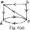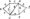# Analysis and Design of Algorithms Jan 2009

PART-A

1 a. Discuss the various stages of algorithm design and analysis process using flow chart.

b. Explain important fundamental problem types of different category.

Explain in brief the basic asymptotic efficiency classes.

2 a.Explain the method of comparing the order of the growth of two functions using limits.

b. Compare order of growth of following functions i) log2 n and Vn ii) ( log2 n)2 and log2 n2 .

3 a. Discuss the general plan for analyzing efficiency of non recursive algorithms.

b. What is brute – force method? Explain sequential search algorithm with an example. Analyse its efficiency.

c. Write the merge sort algorithm and discuss its efficiency. Sort the list E, X, A, M, P, L, E in alphabetical order using merge sort.

4 a. What is divide – and – conquer technique? Apply this method to find multiplication of integers 2101 and 1130.

b. Explain the differences between DFS and BFS. Solve topological sorting problem using DFS algorithm with an example.

PART – B

5 a. Explain bottom – up heap sort algorithm with an example. Analyse its efficiency.

b. Write HorspooFs algorithm.

6 a. Apply Horspool algorithm to search for the pattern BAOBAB in the text BESS_ KNEW_ABOUTJ3AOBABA.b. Write WarshalFs algorithm. Apply WarshalPs algorithm to find the transitive

7 a. Solve the following knapsack problem with given capacity W programming.Item Weight Value 1 2 \$12 2 1 \$10 3 3 \$20 4 2 \$15 apply t le same to find s

= 5 using dynamic

b. Write Dijkstra’s algorithm and apply t: for the following graph taking vertex ‘a’ as source in fig. 7(a).

C. What are decision trees? Explain the concept of decision trees for sorting algorithms with an example.

8 a. Briefly explain the concepts of P5 NP and NP complete problems.

b.        Explain back – tracking algorithm. Apply the same to solve the following instance of the subset – sum problem : S = {3, 5, 6, 7} and d = 15.